Скачать презентацию Optimal-Complexity Optical Router Hadas Kogan Isaac Keslassy Technion

• Количество слайдов: 26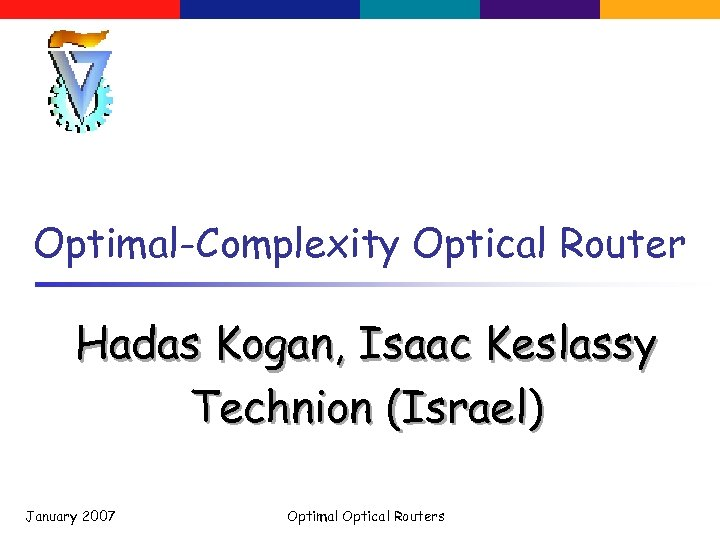Optimal-Complexity Optical Router Hadas Kogan, Isaac Keslassy Technion (Israel) January 2007 Optimal Optical Routers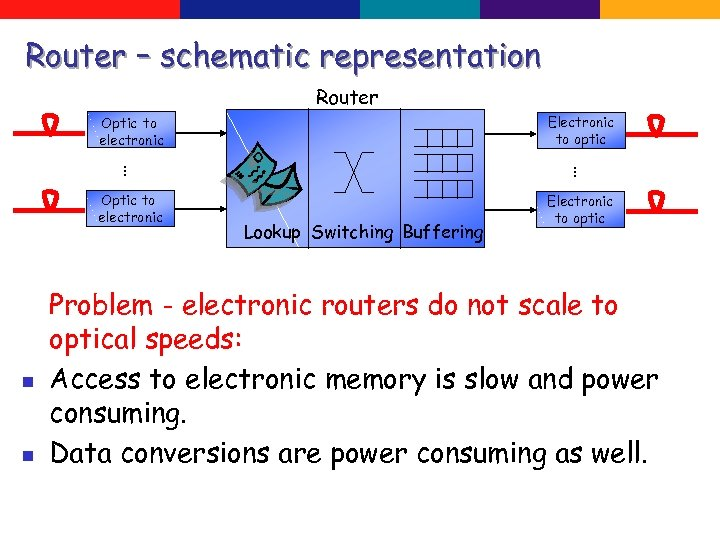Router – schematic representation Router Electronic to optic Optic to electronic … n n … Optic to electronic Electronic to optic Lookup Switching Buffering Problem - electronic routers do not scale to optical speeds: Access to electronic memory is slow and power consuming. Data conversions are power consuming as well.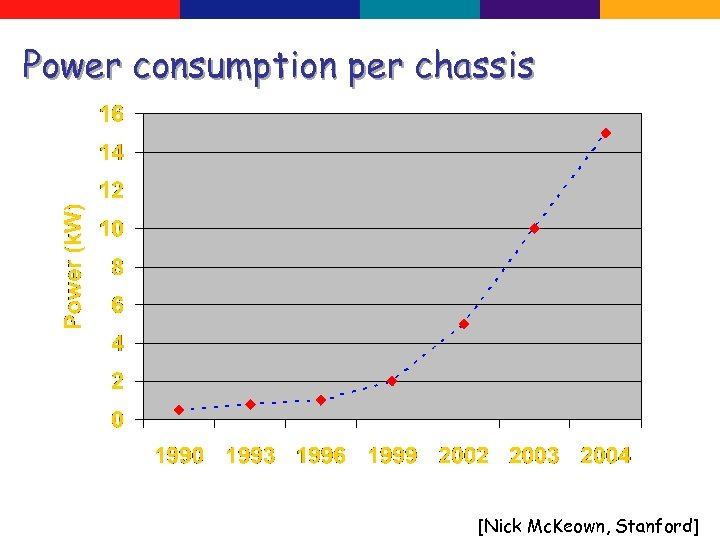Power consumption per chassis [Nick Mc. Keown, Stanford]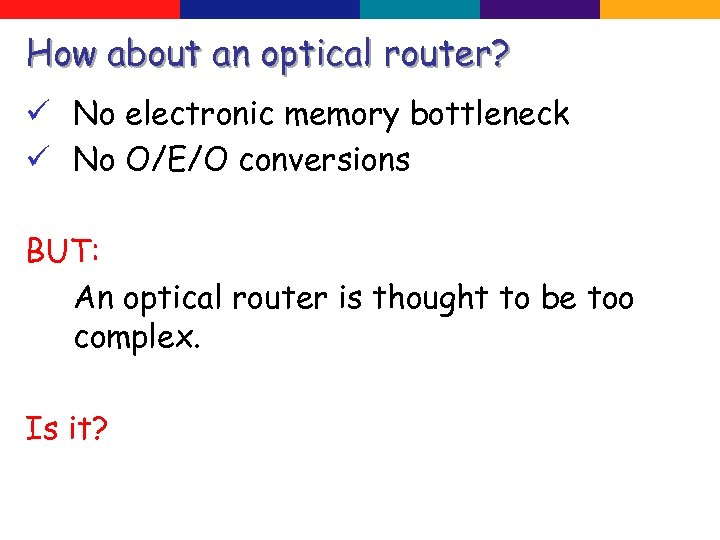How about an optical router? ü No electronic memory bottleneck ü No O/E/O conversions BUT: An optical router is thought to be too complex. Is it?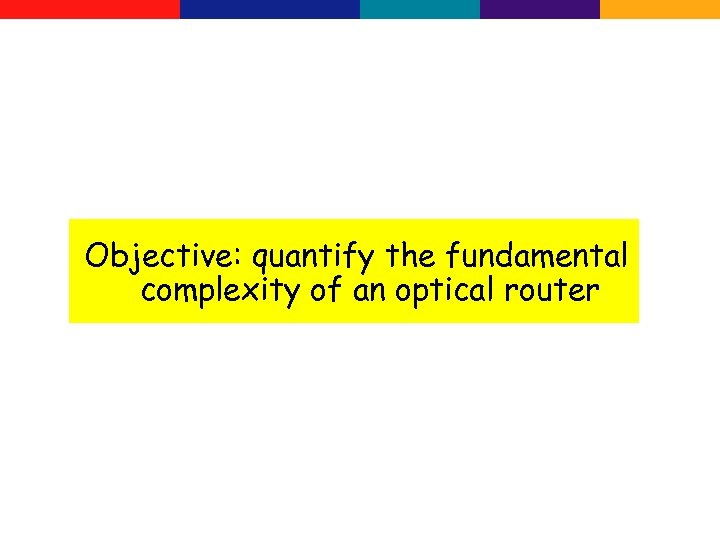Objective: quantify the fundamental complexity of an optical router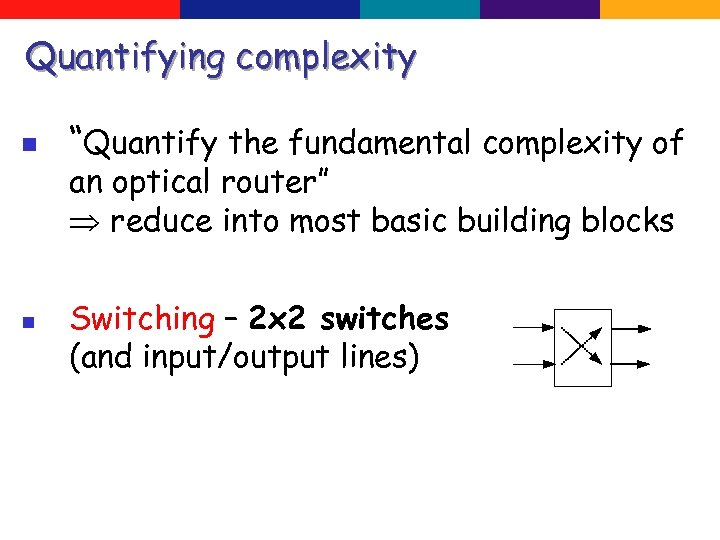Quantifying complexity n n “Quantify the fundamental complexity of an optical router” reduce into most basic building blocks Switching – 2 x 2 switches (and input/output lines)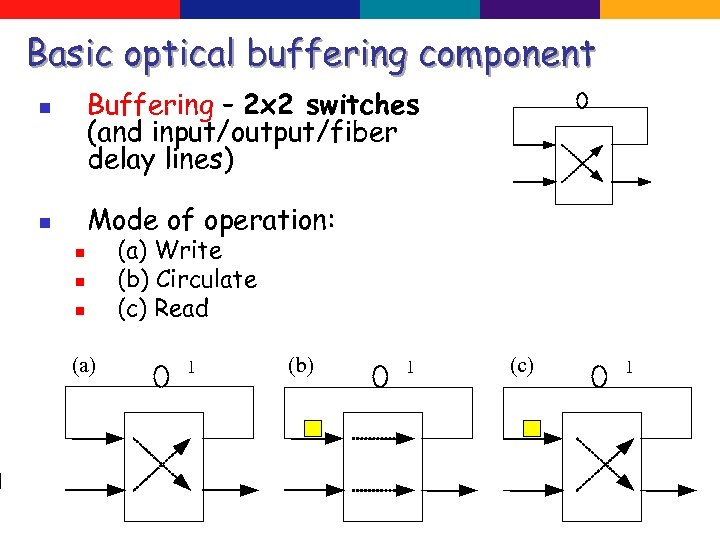Basic optical buffering component Buffering – 2 x 2 switches (and input/output/fiber delay lines) n Mode of operation: n n (a) Write (b) Circulate (c) Read (a) 1 n n (b) 1 (c) 1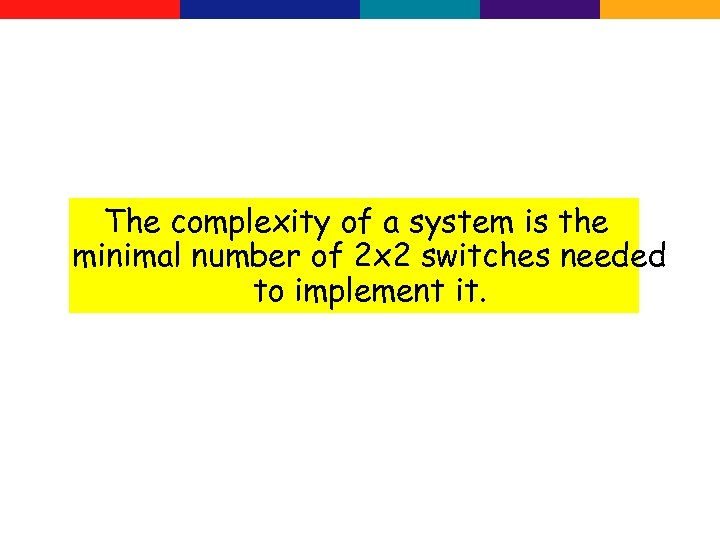The complexity of a system is the minimal number of 2 x 2 switches needed to implement it.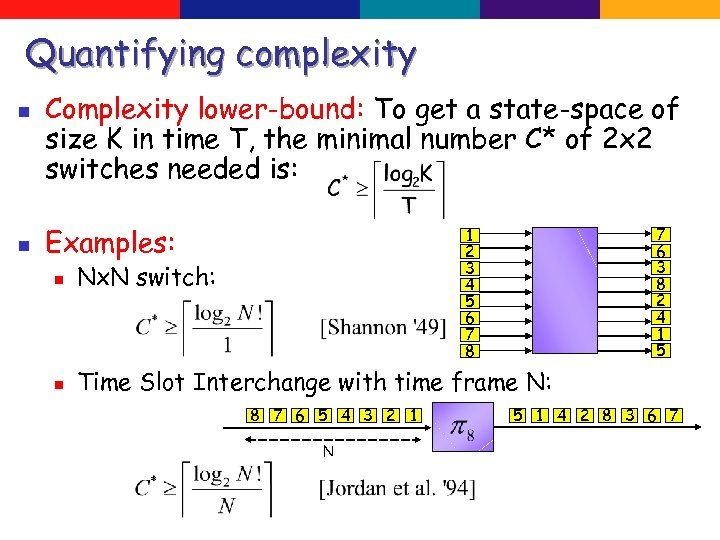Quantifying complexity n n Complexity lower-bound: To get a state-space of size K in time T, the minimal number C* of 2 x 2 switches needed is: Examples: 7 6 3 8 2 4 1 5 1 2 3 4 5 6 7 8 n Nx. N switch: n Time Slot Interchange with time frame N: 8 7 6 5 4 3 2 1 N 5 1 4 2 8 3 6 7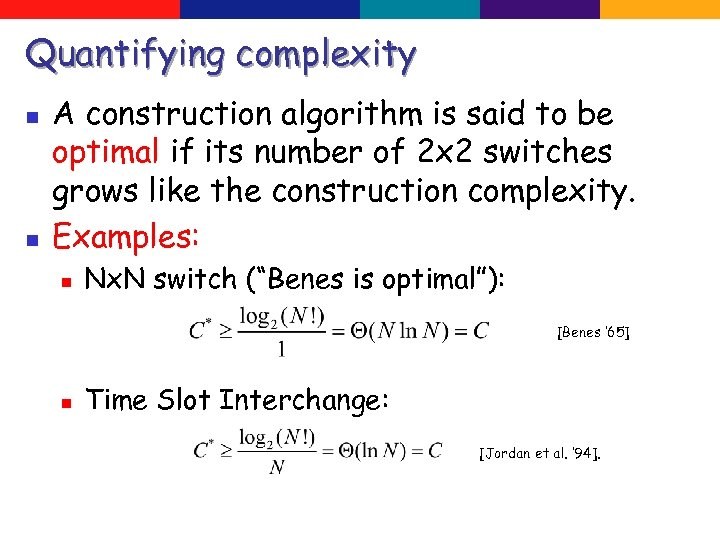Quantifying complexity n n A construction algorithm is said to be optimal if its number of 2 x 2 switches grows like the construction complexity. Examples: n Nx. N switch (“Benes is optimal”): [Benes ‘ 65] n Time Slot Interchange: [Jordan et al. ‘ 94].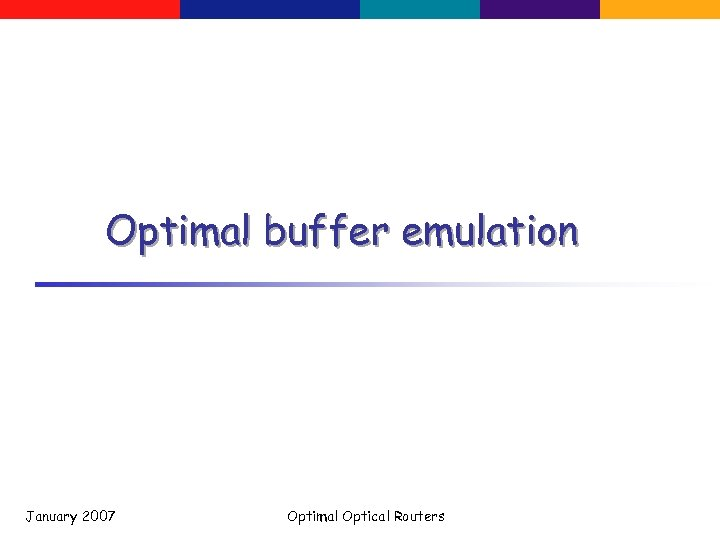Optimal buffer emulation January 2007 Optimal Optical Routers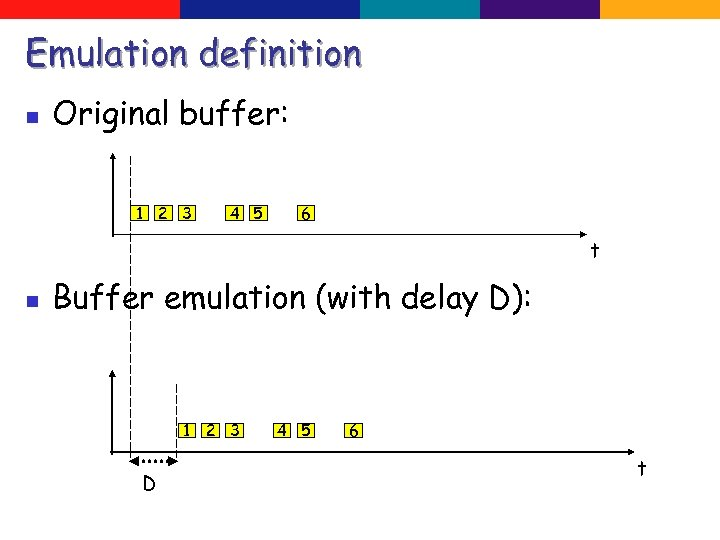Emulation definition n Original buffer: 1 2 3 4 5 6 t n Buffer emulation (with delay D): 1 2 3 D 4 5 6 t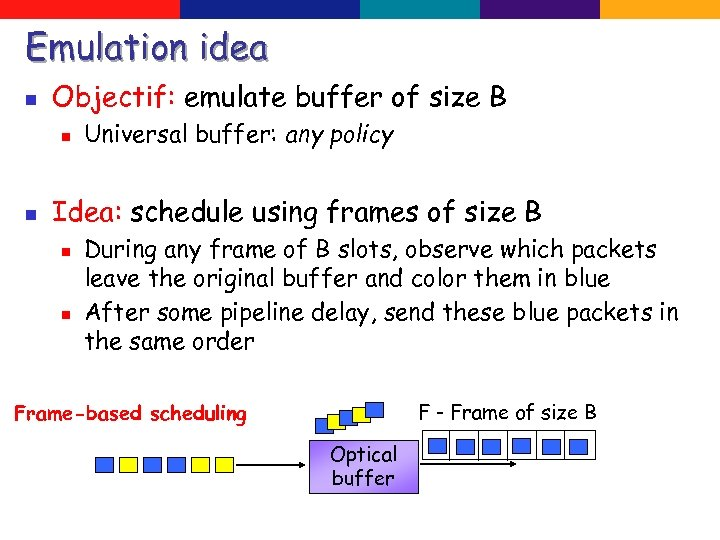Emulation idea n Objectif: emulate buffer of size B n n Universal buffer: any policy Idea: schedule using frames of size B n n During any frame of B slots, observe which packets leave the original buffer and color them in blue After some pipeline delay, send these blue packets in the same order F - Frame of size B Frame-based scheduling Optical buffer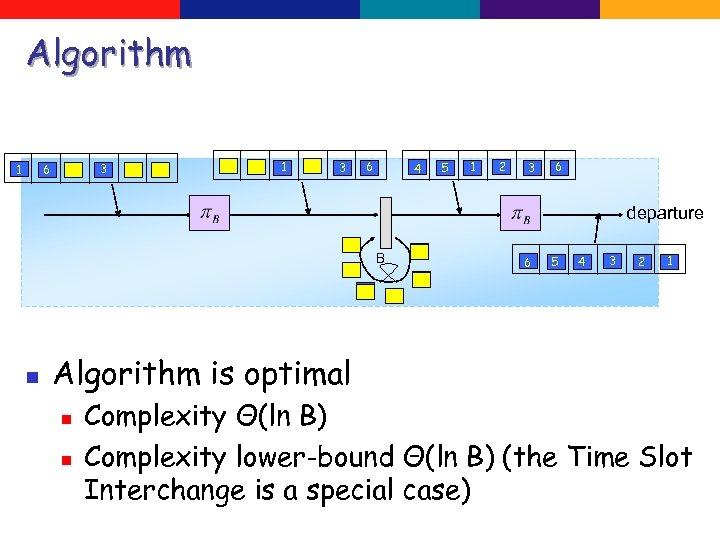Algorithm 3 6 1 1 3 6 4 5 1 2 3 6 departure B 2 n 4 5 6 5 4 3 2 1 Algorithm is optimal n n Complexity Θ(ln B) Complexity lower-bound Θ(ln B) (the Time Slot Interchange is a special case)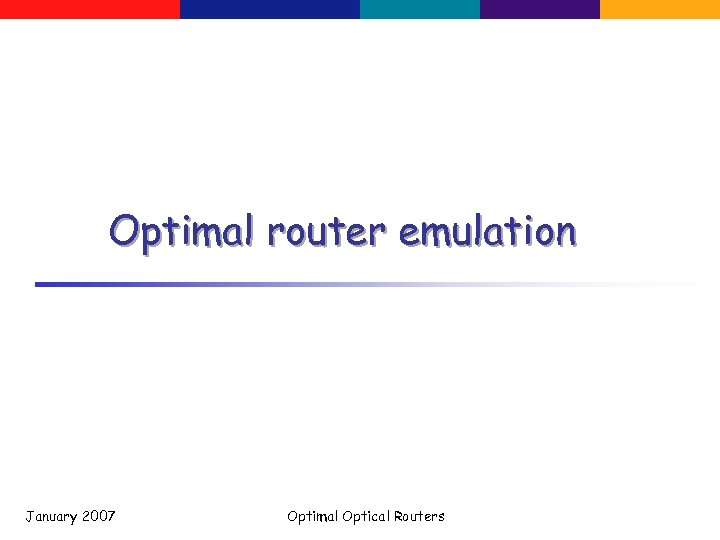Optimal router emulation January 2007 Optimal Optical Routers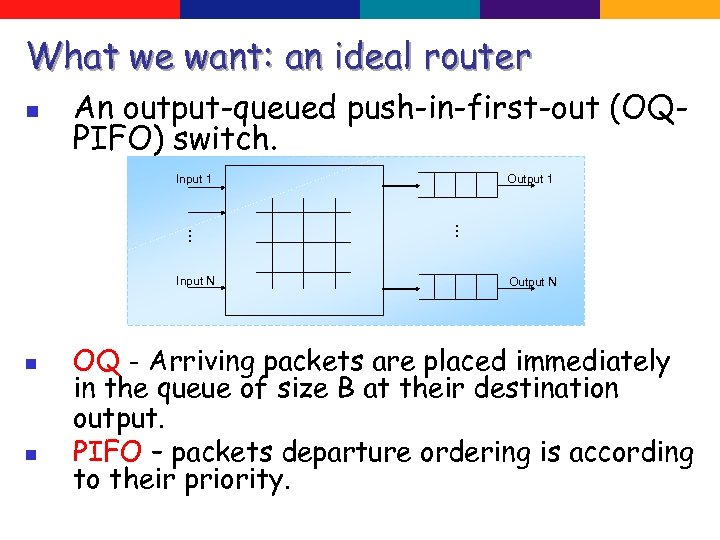What we want: an ideal router n An output-queued push-in-first-out (OQPIFO) switch. Input 1 n n … … Input N Output 1 Output N OQ - Arriving packets are placed immediately in the queue of size B at their destination output. PIFO – packets departure ordering is according to their priority.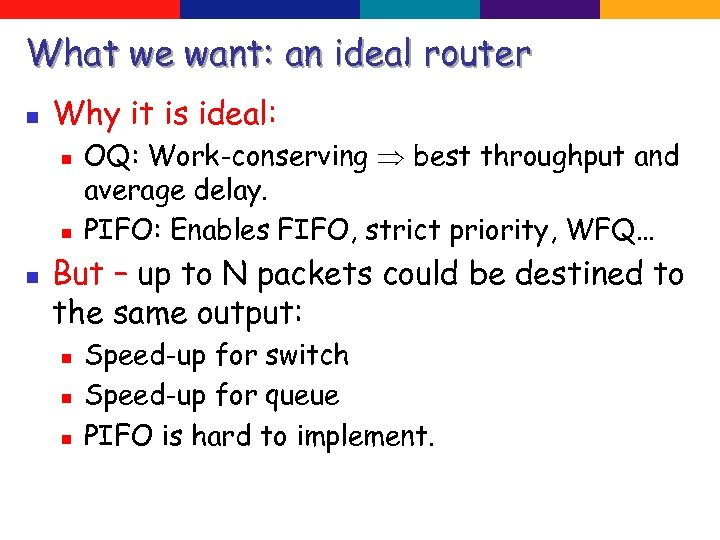What we want: an ideal router n Why it is ideal: n n n OQ: Work-conserving best throughput and average delay. PIFO: Enables FIFO, strict priority, WFQ… But – up to N packets could be destined to the same output: n n n Speed-up for switch Speed-up for queue PIFO is hard to implement.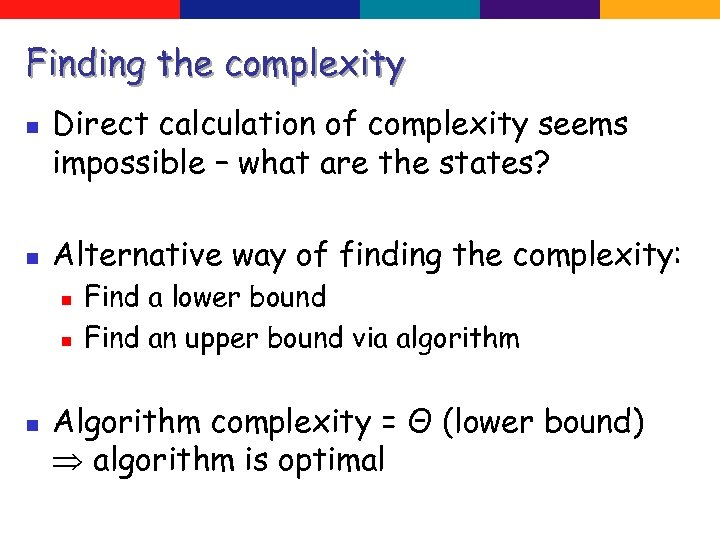Finding the complexity n n Direct calculation of complexity seems impossible – what are the states? Alternative way of finding the complexity: n n n Find a lower bound Find an upper bound via algorithm Algorithm complexity = Θ (lower bound) algorithm is optimal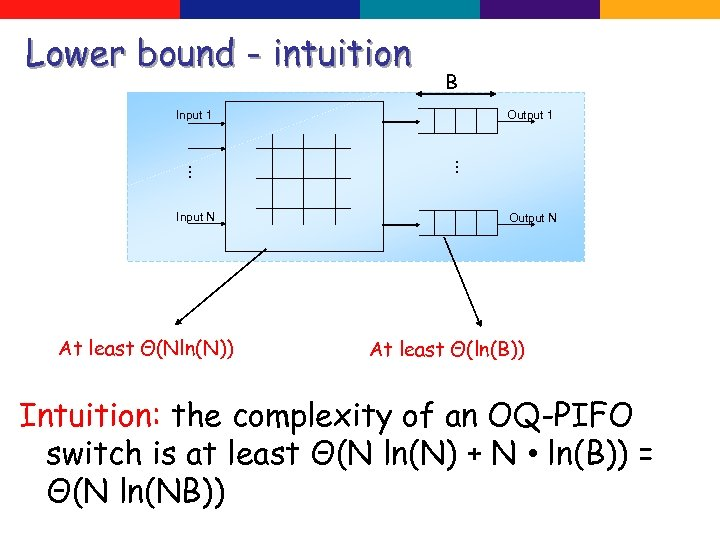Lower bound - intuition B Input 1 At least Θ(Nln(N)) … … Input N Output 1 Output N At least Θ(ln(B)) Intuition: the complexity of an OQ-PIFO switch is at least Θ(N ln(N) + N • ln(B)) = Θ(N ln(NB))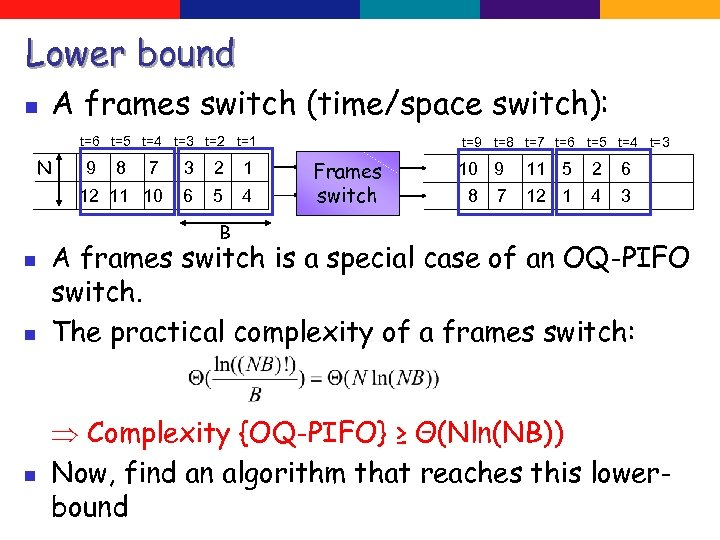Lower bound n A frames switch (time/space switch): t=6 t=5 t=4 t=3 t=2 t=1 N 9 8 7 3 2 1 12 11 10 6 5 4 t=9 t=8 t=7 t=6 t=5 t=4 t=3 Frames switch 10 9 8 7 11 5 2 6 12 1 4 3 B n n n A frames switch is a special case of an OQ-PIFO switch. The practical complexity of a frames switch: Complexity {OQ-PIFO} ≥ Θ(Nln(NB)) Now, find an algorithm that reaches this lowerbound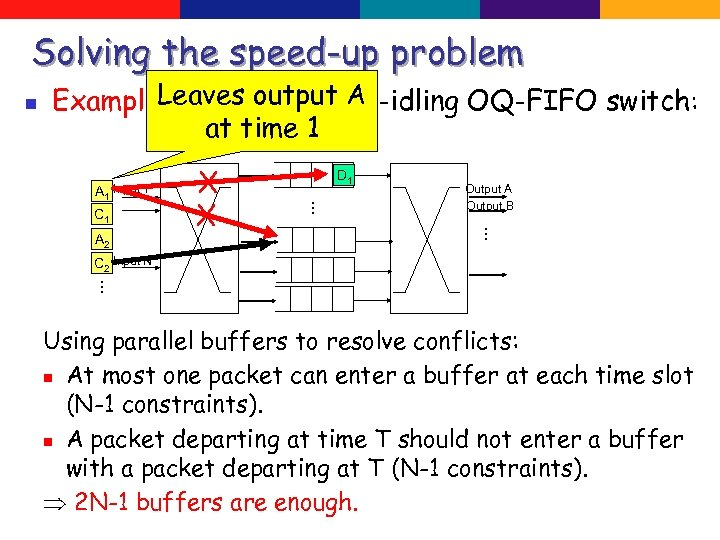Solving the speed-up problem n Leaves output A Example: Emulating a non-idling OQ-FIFO switch: at time 1 C 1 D 1 Output A Output B … A 2 X X … A 1 Input 1 C 2 Input N … Using parallel buffers to resolve conflicts: n At most one packet can enter a buffer at each time slot (N-1 constraints). n A packet departing at time T should not enter a buffer with a packet departing at T (N-1 constraints). 2 N-1 buffers are enough.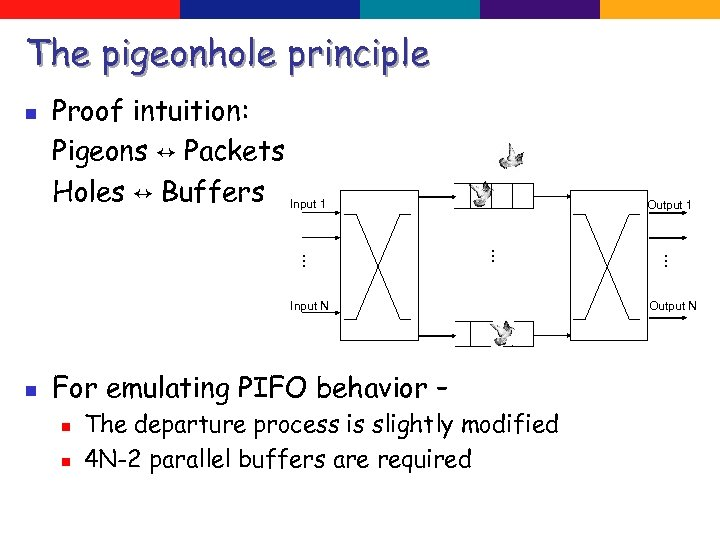The pigeonhole principle n Proof intuition: Pigeons ↔ Packets Holes ↔ Buffers Input 1 Output 1 n For emulating PIFO behavior – n n The departure process is slightly modified 4 N-2 parallel buffers are required … … … Input N Output N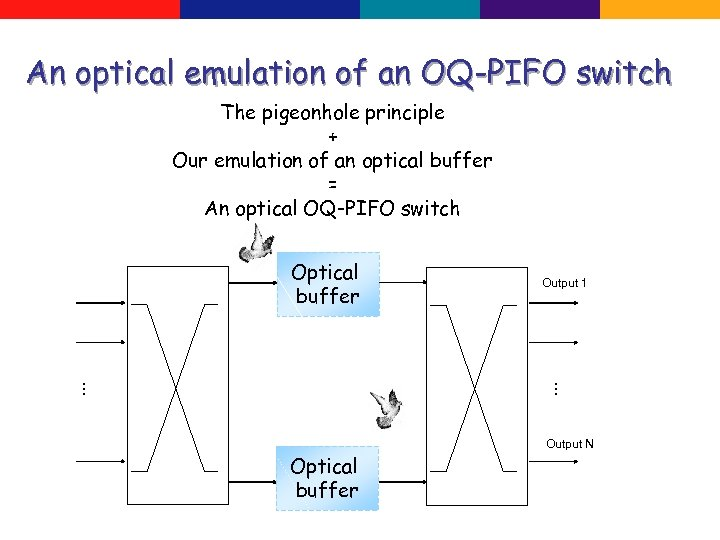An optical emulation of an OQ-PIFO switch The pigeonhole principle + Our emulation of an optical buffer = An optical OQ-PIFO switch Optical buffer Output 1 … … Output N Optical buffer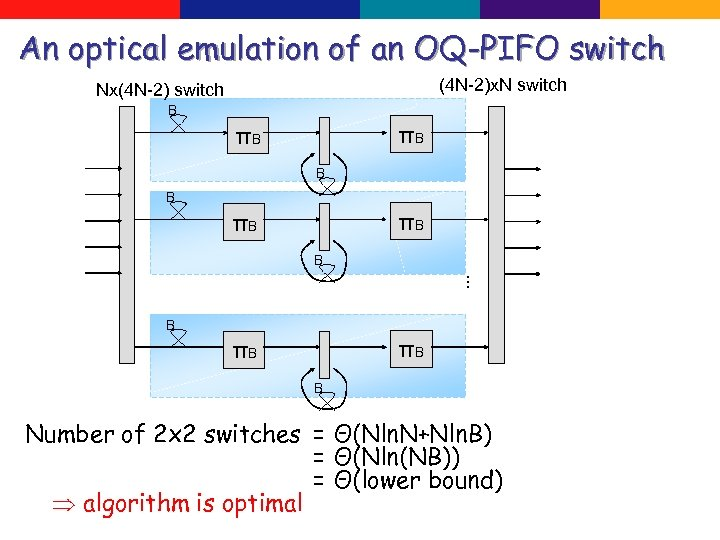An optical emulation of an OQ-PIFO switch (4 N-2)x. N switch Nx(4 N-2) switch B πB πB B . . . B πB πB B Number of 2 x 2 switches = Θ(Nln. N+Nln. B) = Θ(Nln(NB)) = Θ(lower bound) algorithm is optimal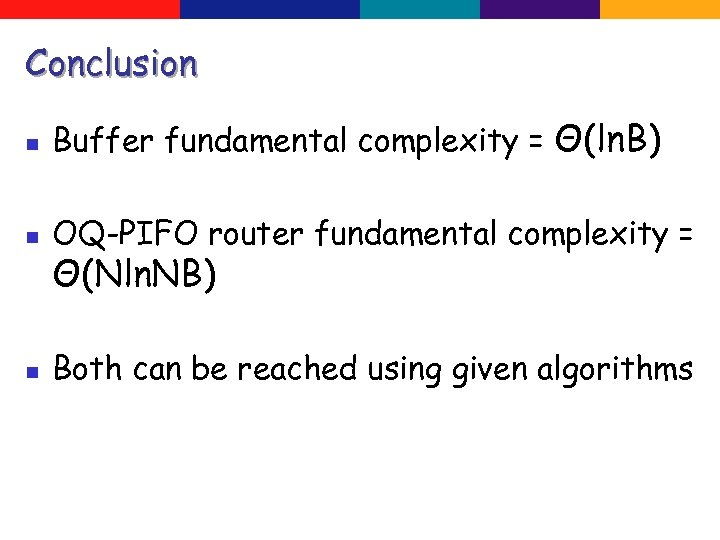Conclusion n Buffer fundamental complexity = Θ(ln. B) n OQ-PIFO router fundamental complexity = n Both can be reached using given algorithms Θ(Nln. NB)Thank you! January 2007 Optimal Optical Routers